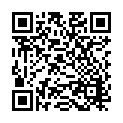Lavoisier S.A.S.
14 rue de Provigny
94236 Cachan cedex
FRANCE

Heures d'ouverture 08h30-12h30/13h30-17h30
Tél.: +33 (0)1 47 40 67 00
Fax: +33 (0)1 47 40 67 02Url canonique : www.lavoisier.fr/livre/mathematiques/patterned-random-matrices/descriptif_3992852
Url courte ou permalien : www.lavoisier.fr/livre/notice.asp?ouvrage=3992852

Patterned Random Matrices

Langue : Anglais

Auteur : Bose Arup• Sommaire

Large dimensional random matrices (LDRM) with specific patterns arise in econometrics, computer science, mathematics, physics, and statistics. This book provides an easy initiation to LDRM. Through a unified approach, we investigate the existence and properties of the limiting spectral distribution (LSD) of different patterned random matrices as the dimension grows. The main ingredients are the method of moments and normal approximation with rudimentary combinatorics for support. Some elementary results from matrix theory are also used. By stretching the moment arguments, we also have a brush with the intriguing but difficult concepts of joint convergence of sequences of random matrices and its ramifications.

This book covers the Wigner matrix, the sample covariance matrix, the Toeplitz matrix, the Hankel matrix, the sample autocovariance matrix and the k-Circulant matrices. Quick and simple proofs of their LSDs are provided and it is shown how the semi-circle law and the Marchenko-Pastur law arise as the LSDs of the first two matrices. Extending the basic approach, we also establish interesting limits for some triangular matrices, band matrices, balanced matrices, and the sample autocovariance matrix. We also study the joint convergence of several patterned matrices, and show that independent Wigner matrices converge jointly and are asymptotically free of other patterned matrices.

Arup Bose is a Professor at the Indian Statistical Institute, Kolkata, India. He is a distinguished researcher in Mathematical Statistics and has been working in high-dimensional random matrices for the last fifteen years. He has been the Editor of Sankyh? for several years and has been on the editorial board of several other journals. He is a Fellow of the Institute of Mathematical Statistics, USA and all three national science academies of India, as well as the recipient of the S.S. Bhatnagar Award and the C.R. Rao Award. His forthcoming books are the monograph, Large Covariance and Autocovariance Matrices (with Monika Bhattacharjee), to be published by Chapman & Hall/CRC Press, and a graduate text, U-statistics, M-estimates and Resampling (with Snigdhansu Chatterjee), to be published by Hindustan Book Agency.

1. A unified framework
2. Empirical and limiting spectral distribution

Moment method

A metric for probability measures

Patterned matrices: A unified approach

Exercises

3. Common symmetric patterned matrices
4. Wigner matrix

Toeplitz and Hankel matrices

Reverse Circulant matrix

Symmetric Circulant and related matrices

Exercises

5. Patterned XXmatrices
6. A unified setup

Aspect ratio y = 0

Aspect ratio y = 0

Exercises

7. Circulant matrices
8. Normal approximation

Circulant matrix

k-Circulant matrices

Exercises

9. Wigner-type matrices
10. Wigner-type matrix

Exercises

11. Balanced Toeplitz and Hankel matrices
12. Main results

Exercises

13. Patterned band matrices
14. LSD for band matrices

Proof

Exercises

15. Triangular matrices
16. General pattern

Triangular Wigner matrix

17. Joint convergence of i.i.d. patterned matrices
18. Non-commutative probability space

Joint convergence

Nature of the limit

Exercises

19. Joint convergence of independent patterned matrices
20. Definitions and notation

Joint convergence

Freeness

Sum of independent patterned matrices

Proofs

Exercises

21. Autocovariance matrix

Preliminaries

Main results

Proofs

Exercises

Date de parution :

15.6x23.5 cm

Disponible chez l'éditeur (délai d'approvisionnement : 13 jours).

Prix indicatif 140,38 €

Mots-clés :

En continuant à naviguer, vous autorisez Lavoisier à déposer des cookies à des fins de mesure d'audience. Pour en savoir plus et paramétrer les cookies, rendez-vous sur la page Confidentialité & Sécurité.
FERMER